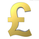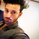32625 views
32625
```//╭╮╱╱╭╮╭╮╱╱╭╮
//┃╰╮╭╯┃┃┃╱╱┃┃
//╰╮┃┃╭┻╯┣╮╭┫╰━┳╮╭┳━━╮
//╱┃╰╯┃╭╮┃┃┃┃╭╮┃┃┃┃━━┫
//╱╰╮╭┫╰╯┃╰╯┃╰╯┃╰╯┣━━┃
//╱╱╰╯╰━━┻━━┻━━┻━━┻━━╯
//╭━━━┳╮╱╱╱╱╱╱╱╭╮
//┃╭━╮┃┃╱╱╱╱╱╱╱┃┃
//┃┃╱╰┫╰━┳━━┳━╮╭━╮╭━━┫┃
//┃┃╱╭┫╭╮┃╭╮┃╭╮┫╭╮┫┃━┫┃
//┃╰━╯┃┃┃┃╭╮┃┃┃┃┃┃┃┃━┫╰╮
//╰━━━┻╯╰┻╯╰┻╯╰┻╯╰┻━━┻━╯
//━╯
//Vdub Renko SniperVX1 v1 // ATR Setting = 1
study("Vdub Renko SniperVX1 v1", overlay=true, shorttitle="Vdub_Renko_SniperVX1_v1")
//===================Candle body resistance Channel==================//
len = 34
src = input(close, title="Renko Channel")
out = sma(src, len)
last8h = highest(close, 13)
lastl8 = lowest(close, 13)
bearish = cross(close,out) == 1 and falling(close, 1)
bullish = cross(close,out) == 1 and rising(close, 1)
channel2=input(true, title="Renko Channel On/Off")
ul2=plot(channel2?last8h:last8h==nz(last8h)?last8h:na, color=black, linewidth=1, style=linebr, title="Renko Channel top", offset=0)
ll2=plot(channel2?lastl8:lastl8==nz(lastl8)?lastl8:na, color=black, linewidth=1, style=linebr, title="Renko Channel bottom", offset=0)
fill(ul2, ll2, color=fuchsia, transp=90, title="Renko Channel")
//=============================================================//

// Moddified [RS]Support and Resistance V0
RST = input(title='Renko Channel length', type=integer, defval=1)     // color zone length
RSTT = valuewhen(high >= highest(high, RST), high, 0)
RSTB = valuewhen(low <= lowest(low, RST), low, 0)
RT2 = plot(RSTT, color=RSTT != RSTT ? na : red, linewidth=1, offset=+0)
RB2 = plot(RSTB, color=RSTB != RSTB ? na : green, linewidth=1, offset=0)

//--------------------Trend colour ema------------------------------------------------//
src0 = close, len0 = input(5, minval=1, title="EMA 1")
ema0 = ema(src0, len0)
direction = rising(ema0, 2) ? +1 : falling(ema0, 2) ? -1 : 0
plot_color = direction > 0  ? lime: direction < 0 ? red : na
plot(ema0, title="EMA", style=line, linewidth=1, color = plot_color)
//--------------------Trend colour ema 2------------------------------------------------//
src02 = close, len02 = input(8, minval=1, title="EMA 2")
ema02 = ema(src02, len02)
direction2 = rising(ema02, 2) ? +1 : falling(ema02, 2) ? -1 : 0
plot_color2 = direction2 > 0  ? lime: direction2 < 0 ? red : na
plot(ema02, title="EMA Signal 2", style=line, linewidth=1, color = plot_color2)
//
//--Modified vyacheslav.shindin-------------------------------------------------//           Signal 1
//Configured ema signal output
fast = input(5, minval=1, title="Short Signal Generator")
slow = input(8, minval=1)
vh1 = ema(highest(avg(low, close), fast), 5)
vl1 = ema(lowest(avg(high, close), slow), 8)
//
e_ema1 = ema(close, 1)
e_ema2 = ema(e_ema1, 1)
e_ema3 = ema(e_ema2, 1)
tema = 1 * (e_ema1 - e_ema2) + e_ema3
//
e_e1 = ema(close, 8)
e_e2 = ema(e_e1, 5)
dema = 2 * e_e1 - e_e2
signal = tema > dema ? max(vh1, vl1) : min(vh1, vl1)
is_call = tema > dema and signal > low and (signal-signal > signal-signal)
is_put = tema < dema and signal < high and (signal-signal > signal-signal)
plotshape(is_call and direction > 0 ? 1 : na, title="BUY ARROW", color=green, text="*BUY*", style=shape.arrowup, location=location.belowbar)
plotshape(is_put and direction < 0 ? -1 : na, title="SELL ARROW", color=red, text="*SELL*", style=shape.arrowdown)
//
//Modified - Rajandran R Supertrend----------------------------------------------------- //       Signal 2
Factor=input(1, minval=1,maxval = 1000, title="Trend Transition Signal")
Pd=input(1, minval=1,maxval = 1000, title="Period")
Up=hl2-(Factor*atr(Pd))
Dn=hl2+(Factor*atr(Pd))
TrendUp=close>TrendUp? max(Up,TrendUp) : Up
TrendDown=close<TrendDown? min(Dn,TrendDown) : Dn
Trend = close > TrendDown ? 1: close< TrendUp? -1: nz(Trend,0)
plotarrow(Trend == 1 and Trend == -1 ? Trend : na, title="Up Entry Arrow", colorup=lime, maxheight=1000, minheight=50, transp=85)
plotarrow(Trend == -1 and Trend == 1 ? Trend : na, title="Down Entry Arrow", colordown=red, maxheight=1000, minheight=50, transp=85)
//
//===============================Directional Projection=======================================//
//=============Hull MA//
show_hma = input(true, title="Display Hull MA Set:")
hma_src = input(close, title="Hull MA's Source:")
hma_base_length = input(8, minval=1, title="Hull MA's Base Length:")
hma_length_scalar = input(5, minval=0, title="Hull MA's Length Scalar:")
hullma(src, length)=>wma(2*wma(src, length/2)-wma(src, length), round(sqrt(length)))
plot(not show_hma ? na : hullma(hma_src, hma_base_length+hma_length_scalar*6), color=black, linewidth=3, title="Hull MA")
//===============================================
//
fill(ul2, RT2, color=red, transp=75, title="Fill")
fill(ll2, RB2, color=green, transp=75, title="Fill")
////////////////////////////////////////////////////////////////////////////////////////////```Is there a way of setting alerts using the Trading View Alerts function so that an alert is triggered when either a BUY ARROW or SELL ARROW is printed on the chart? Otherwise the script is only good for day trading as the trader would need to be at the screen constantly watching it so as not to miss an entry or exit.

I've tried every setting I can think of for it, and can't get an alert to trigger for the above :-(hi,

I used VDUB RENKO SNIPER VX1 V1 for Binary Option 60 Sec Trading, Following is the strategy I used with result

I conduct a test for 1 hour using VDUB RENKO SNIPER VX1 V1

|||| STRATEGY:

Whole concept of this strategy is following Trend,

1) On 1 min Chart

Look for a Trend following with a large arrow, Lets take example of a down trend

2) Look for Down Trend
3) Wait for SELL signal

In this stage do not SELL until you see a SELL signal even though you will see continues down trend still don't SELL until you see a SELL signal whole concept of this strategy is to be on Safe side and follow trend.

While checking for SELL signal you might see some BUY signal even though the TREND is DOWN, just ignore them as you are currently looking for DOWN TREND / SELL SIGNALS.

4) PUT once you see a SELL signal ( Idol time for placing Trade would be 20-10 prior to PURCHASE TIME)

So yeah this is the whole strategy I use to check this indicator for BINARY OPTION 60 SEC TRADING.

||| RESULTS:
=> Wins: 14
=> Loss: 8

85% Was the Winning profit

=> Consecutive Loss: 1

This strategy is still in profit but not as much as it should be But the thing that should be noticed is the Consecutive loss figure, which is just 1 so maybe if we double up the amount when we lose the trade there is possibility of getting higher payout.

I haven't tried it yet, Maybe by tomorrow, ill test and post it here. the other thing is I couldnt calculate the profit, lol not really good in math, each trade was of \$10 and winning amount per winning trade was 85% then according to my above winning ratio can any body demonstrate the profit?